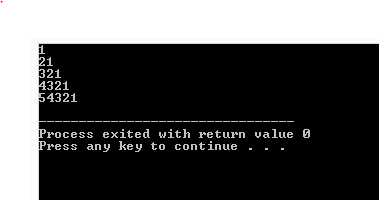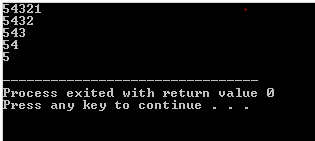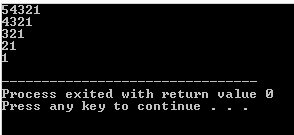## Posts

### Print pattern in c - number pattern -8Print pattern in c - number pattern Write a program to print following pattern 5 54 543 5432 54321 Program code #include <stdio.h> int main() { int i, j; for(i=5;i>=1;i--) { for(j=5;j>=i;j--) { printf("%d",j); } printf("\n"); } return 0; } Step by Step solution 1. Number of row -    Is has 5row  , so first loop will execute for 5 time #include <stdio.h> int main() {     int i, j;     for(i=5;i>=1;i--)     {...... }     return 0; } 2. Number of character -  It has only one type of character , so there will be only one nested loop #include <stdio.h> int main() { ...... for(j=5;j>=i;j--) { printf("%d",j); } printf("\n"); } 3.Number of column - It has 5 maximum column and is decreasing with row number , so the nested loop decrease it's repetation with increase in row #i

### Print pattern in c - number pattern-7Print pattern in c - number pattern Write a program to print following pattern 1 21 321 4321 54321 Program code #include <stdio.h> int main() { int i, j; for(i=1;i<=5;i++) { for(j=i;j>=1;j--) { printf("%d",j); } printf("\n"); } return 0; } Step by Step solution 1. Number of row -    Is has 5row  , so first loop will execute for 5 time #include <stdio.h> int main() {     int i, j;     for(i=1;i<=5;i++)     {...... }     return 0; } 2. Number of character -  It has only one type of character , so there will be only one nested loop #include <stdio.h> int main() { ...... for(j=i;j>=1;j--) { printf("%d",j); } printf("\n"); } 3.Number of column - It has 5 maximum column and is decreasing with row number , so the nested loop decrease it's repetation with increase in row #i

### Print pattern in c - number pattern -6Print pattern in c - number pattern -6 Write a program to print following pattern 5 45 345 2345 12345 Program code #include <stdio.h> int main() { int i, j; for(i=5;i>=1;i--) { for(j=i;j<=5;j++) { printf("%d",j); } printf("\n"); } return 0; } Step by Step solution 1. Number of row -    Is has 5row  , so first loop will execute for 5 time #include <stdio.h> int main() {     int i, j;     for(i=5;i>=1;i--)     {...... }     return 0; } 2. Number of character -  It has only one type of character , so there will be only one nested loop #include <stdio.h> int main() { ...... for(j=i;j<=5;j++) { printf("%d",j); } printf("\n"); } 3.Number of column - It has 5 maximum column and is decreasing with row number , so the nested loop decrease it's repetation with increase in row #i

### Print pattern in c - number pattern -5Print pattern in c - number pattern -5 Write a program to print following pattern 1 12 123 1234 12345 Program code #include <stdio.h> int main() { int i, j; for(i=1;i<=5;i++) { for(j=1;j<=i;j++) { printf("%d",j); } printf("\n"); } return 0; } Step by Step solution 1. Number of row -    Is has 5row  , so first loop will execute for 5 time #include <stdio.h> int main() {     int i, j;     for(i=1;i<=5;i++)     {...... }     return 0; } 2. Number of character -  It has only one type of character , so there will be only one nested loop #include <stdio.h> int main() { ...... for(j=1;j<=i;j++) { printf("%d",j); } printf("\n"); } 3.Number of column - It has 5 maximum column and is decreasing with row number , so the nested loop decrease it's repetation with increase in row

### Print pattern in c - number pattern -4Print pattern in c - number pattern -4 Write a program to print following pattern 54321 5432 543 54 5 Program code #include <stdio.h> int main() { int i, j; for(i=1;i<=5;i++) { for(j=5;j>=i;j--) { printf("%d",j); } printf("\n"); } return 0; } Step by Step solution 1. Number of row -    Is has 5row  , so first loop will execute for 5 time #include <stdio.h> int main() {     int i, j;     for(i=1;i<=5;i++)     {...... }     return 0; } 2. Number of character -  It has only one type of character , so there will be only one nested loop #include <stdio.h> int main() { ...... for(j=5;j>=i;j--) { printf("%d",j); } printf("\n"); return 0; } 3.Number of column - It has 6 maximum column and is decreasing with row number , so the nested loop decrease it's repetation with

### Print pattern in c - number pattern -3Print pattern in c - number pattern -3 Write a program to print following pattern 54321 4321 321 21 1 Program code #include <stdio.h> int main() { int i, j; for(i=5;i>=1;i--) { for(j=i;j>=1;j--) { printf("%d",j); } printf("\n"); } return 0; } Step by Step solution 1. Number of row -    Is has 5row  , so first loop will execute for 5 time #include <stdio.h> int main() {     int i, j;     for(i=5;i>=1;i--)     {...... }     return 0; } 2. Number of character -  It has only one type of character , so there will be only one nested loop #include <stdio.h> int main() { ...... for(j=i;j>=1;j--) { printf("%d",j); } printf("\n"); } 3.Number of column - It has 5 maximum column and is decreasing with row number , so the nested loop decrease it's repetation with increase in row #include <stdio.h>

### Print pattern in c - number pattern -2

Print pattern in c - number pattern -2 Write a program to print following pattern 12345 2345 345 45 5 Program code #include <stdio.h> int main() {     int i, j;     for(i=1;i<=5;i++)     {         for(j=i;j<=5;j++)         {             printf("%d",j);         }         printf("\n");     }     return 0; } Step by Step solution 1. Number of row -    Is has 5row  , so first loop will execute for 5 time #include <stdio.h> int main() {     int i, j;     for(i=1;i>=5;i++)     {...... }     return 0; } 2. Number of character -  It has only one type of character , so there will be only one nested loop #include <stdio.h> int main() { ...... for(j=i;j<=5;j++)         {             printf("%d",j);         }         printf("\n"); return 0; } 3.Number of column - It has 6 maximum column and is decreasing with row number , so the nested loop decrease it's repetation with increase